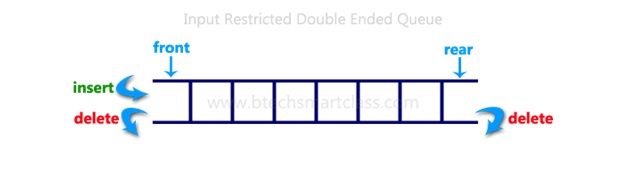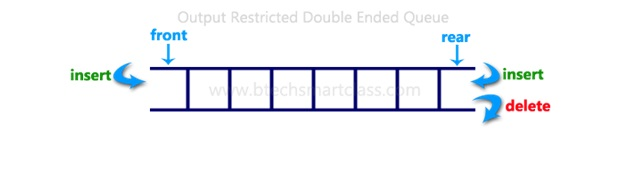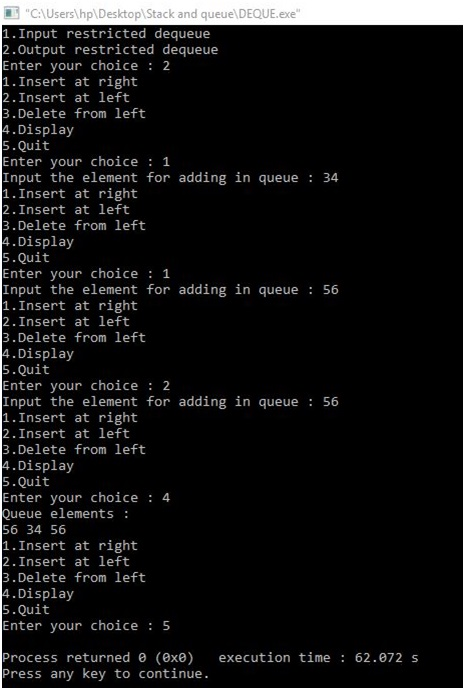# Implementation of Deque using Array

In this article, we are going to learn how to create an input and output restricted Deque with the help of array in the data structure?
Submitted by Manu Jemini, on December 19, 2017

This differs from the queue abstract data type or First-In-First-Out List (FIFO), where elements can only be added to one end and removed from the other. This general data class has some possible sub-types:

1. An input-restricted Deque is one where deletion can be made from both ends, but insertion can be made at one end only.
2. An output-restricted Deque is one where insertion can be made at both ends, but deletion can be made from one end only.

Input Restricted DequeOutput Restricted DequeImage source: http://btechsmartclass.com/DS/images/

The Below code consists of many functions. Four functions are general, two display functions, two special and the main function.

The implementation starts with the main function and then user choose input or output type of restricted queues. According to the choice, one of the two special functions gets invoked and that function leads the way.

As above explained a little about the operational rules of both types, the user gets the options to operate.

There are four functions insert_left, insert_right, delete_left and delete_right. As the naming specify these functions add or delete to the corresponding sides.

Then we got two display functions for both the different type types of a queue. The Size of array is 5 by default, to change, edit the second line of code.

## C program to implement DeQue using Array

```# include<stdio.h>
# define MAX 5

int deque_arr[MAX];
int left = -1;
int right = -1;

/*Begin of insert_right*/
void insert_right()
{
if((left == 0 && right == MAX-1) || (left == right+1))
{	printf("Queue Overflow\n");
return;}
if (left == -1)  /* if queue is initially empty */
{	left = 0;
right = 0;}
else
if(right == MAX-1)  /*right is at last position of queue */
right = 0;
else
right = right+1;
printf("Input the element for adding in queue : ");
}
/*End of insert_right*/

/*Begin of insert_left*/
void insert_left()
if((left == 0 && right == MAX-1) || (left == right+1))
{	printf("Queue Overflow \n");
return;	 }
if (left == -1)/*If queue is initially empty*/
{	left = 0;
right = 0;	 }
else
if(left== 0)
left=MAX-1;
else
left=left-1;
printf("Input the element for adding in queue : ");
/*End of insert_left*/

/*Begin of delete_left*/
void delete_left()
{	if (left == -1)
{	printf("Queue Underflow\n");
return ;	}
printf("Element deleted from queue is : %d\n",deque_arr[left]);
if(left == right) /*Queue has only one element */
{	left = -1;
right=-1;	 }
else
if(left == MAX-1)
left = 0;
else
left = left+1;
}
/*End of delete_left*/

/*Begin of delete_right*/
void delete_right()
{if (left == -1)
{printf("Queue Underflow\n");
return ;	 }
printf("Element deleted from queue is : %d\n",deque_arr[right]);
if(left == right) /*queue has only one element*/
{	left = -1;
right=-1;	 }
else
if(right == 0)
right=MAX-1;
else
right=right-1;	}
/*End of delete_right*/
/*Begin of input_que*/
void display_queue()
{	int front_pos = left,rear_pos = right;
if(left == -1)
{	printf("Queue is empty\n");
return;	 }
printf("Queue elements :\n");
if( front_pos <= rear_pos )
{	while(front_pos <= rear_pos)
{	printf("%d ",deque_arr[front_pos]);
front_pos++;	}	}
else
{	while(front_pos <= MAX-1)
{	printf("%d ",deque_arr[front_pos]);
front_pos++;	}
front_pos = 0;
while(front_pos <= rear_pos)
{	printf("%d ",deque_arr[front_pos]);
front_pos++;
}
}
printf("\n");
}
/*End of display_queue*/
/*Begin of input_que*/
void input_que()
{	int choice;
do
{	printf("1.Insert at right\n");
printf("2.Delete from left\n");
printf("3.Delete from right\n");
printf("4.Display\n");
printf("5.Quit\n");
scanf("%d",&choice);

switch(choice)
{	case 1:
insert_right();
break;
case 2:
delete_left();
break;
case 3:
delete_right();
break;
case 4:
display_queue();
break;
case 5:
break;
default:
printf("Wrong choice\n");
}
}while(choice!=5);
}
/*End of input_que*/

/*Begin of output_que*/
void output_que()
{	int choice;
do
{	printf("1.Insert at right\n");
printf("2.Insert at left\n");
printf("3.Delete from left\n");
printf("4.Display\n");
printf("5.Quit\n");
scanf("%d",&choice);
switch(choice)
{
case 1:
insert_right();
break;
case 2:
insert_left();
break;
case 3:
delete_left();
break;
case 4:
display_queue();
break;
case 5:
break;
default:
printf("Wrong choice\n");
}
}while(choice!=5);
}
/*End of output_que*/

/*Begin of main*/
main()
{	int choice;
printf("1.Input restricted dequeue\n");
printf("2.Output restricted dequeue\n");
scanf("%d",&choice);
switch(choice)
{
case 1 :
input_que();
break;
case 2:
output_que();
break;
default:
printf("Wrong choice\n");
}
}
/*End of main*/
```

Output